# Operations Of Integers

Operations of integers will include the basic arithmetic operations (addition, subtraction, multiplication and division). In Maths, integers are the numbers that include all the positive numbers, negative numbers and zero, excluding fractions. Hence, it is easy to perform operations on integers. We will discuss here integers operations on a number line with examples.

## Operations of Integers on a number line

A number line is a reference for comparing and ordering numbers. Every number on the number line is plotted with respect to the origin (zero), and the position of a number on a number line determines the value of that number. This implies that numbers right to zero are known as positive numbers, and the numbers left to zero are known as negative numbers.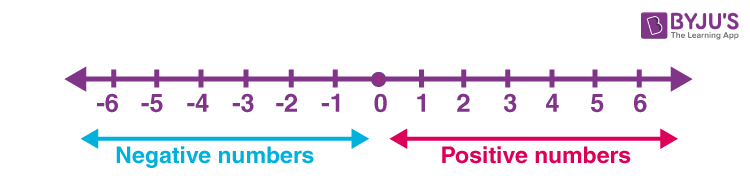## Addition and Subtraction of Integers on Number Line

Arithmetic operations on integers can be better explained on a number line. To begin with, one must know to locate integers on a number line. Zero is the middle point of a number line. All positive numbers occupy the right side of zero, whereas negative numbers occupy the left side of zero.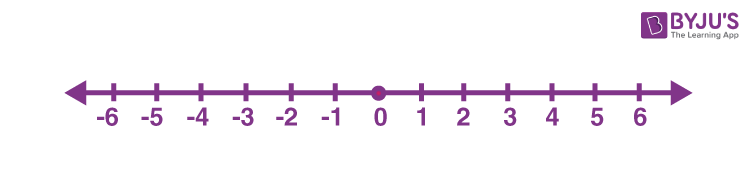Whether it is the addition or subtraction of two integers, begin with the first number. Locate the first number on the number line. The first number lets us know where to begin. The second number lets know the direction of movement. If it is a positive number, move to the right side by that many units and if it is a negative number, move to the left side of the first number by that many units.

Positive numbers:  When we add two positive numbers, the result will always be a positive number. Hence, on adding positive numbers, the direction of the move will always be to the right side.

For example, addition of 1 and 5 (1 + 5 = 6)

Here the first number is 1, and the second number is 5; both are positive. First, locate 1 on a number line. Then moving 5 places to the right will give 6.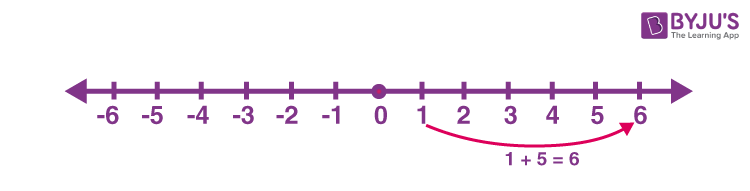Negative numbers:  When we add two negative numbers, the result will always be a negative number. Hence, on adding negative numbers, the direction of the move will always be to the left side.

For example, the addition of -2 and -3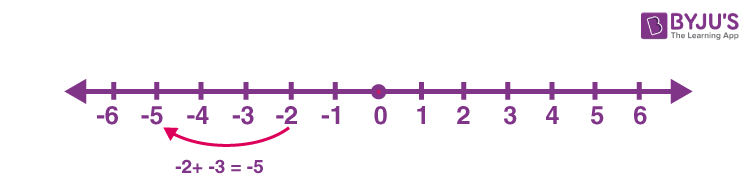Here the first number is -2, and the second number is -3; both are negative. Locate -2 on a number line. Then moving 3 places to the left will give -5.

### Subtraction of Integers

Positive numbers:  When we subtract two positive numbers, move to the left as far as the value of the second number.

For example, subtract 5 from 2

Here the first number is 2, and the second number is 5; both are positive. First, locate 2 on a number line. Then moving 5 places to the left will give -3.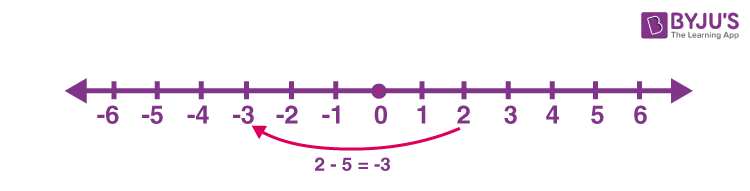Negative numbers:  When we subtract two negative numbers, move to the right as far as the value of the second number.

For example, subtract -4 from -2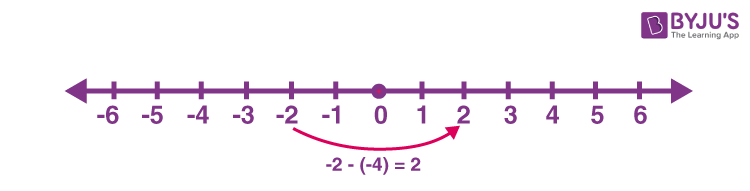First, locate -2 on a number line. Then moving 4 places to the right will give 2.

## Multiplication and Division of Integers

It is difficult to show the multiplication and division operations of integers on a number line. It is because the multiplication of integers leads to larger values and the division of integers may result in fractions. Let us learn multiplication and division operations performed on integers.

### Multiplication of Integers

When two integers are multiplied then the product is an integer only. The rules of multiplication of integers are as follows:

• Product of two positive integers is positive (Eg. 2 × 3 = 6)
• Product of two negative integers is negative (Eg. -3 × -4 = 12)
• Product of a positive and a negative integer is negative (Eg. -3 × 5 = -15)

To sum up, we have:

 (+ve) × (+ve) (+ve) (-ve) × (-ve) (+ve) (-ve) × (+ve) (-ve) (+ve) × (-ve) (-ve)

### Division of Integers

When one integer is divided by another integer then the product is either an integer or a fraction. The rules of division of integers are as follows:

• Division of two positive integers results in a positive value (Eg. 9 ÷ 3 = 3)
• Division of two negative integers results in a negative value (Eg. -4 ÷ -2 = 2)
• Division of a positive and a negative integer or vice-versa results in a negative value (Eg.  -10 ÷ 5 = -2)

To sum up with the rules, we have:

 (+ve) ÷ (+ve) (+ve) (-ve) ÷ (-ve) (+ve) (-ve) ÷ (+ve) (-ve) (+ve) ÷ (-ve) (-ve)

## Rules of Operation With Integers

The rules of operations on integers are listed below:

• Addition of two integers with the same sign will result in the sum of integers with the same sign.
• Addition of two integers with different signs will result in the difference of integers with the sign of greater value
• Subtraction of integers will follow similar rules as an addition. One important rule is the sign of integer, which is subtracted from other integers, changed and added to the other integer.
• Multiplication and division of two same-sign integers will result in a positive value
• Multiplication and division of two different sign integers will result in a negative value

## Solved Examples – Operations of Integers

Solve the following operations of integers.

1. 20 + 30 = ?
2. -10 – 13 = ?
3. -9 + 3 = ?
4. 10 – 4 = ?
5. (+3) x (-1) = ?
6. (+7) x (+6) = ?
7. (-5) x (-5) = ?
8. (-8) x (-6) = ?
9. (+9) ÷ (-3) = ?
10. (+15) ÷ (5) = ?

Solution:

1. 20 + 30 = 50
2. -10 – 13 = -23
3. -9 + 3 = -6
4. 10 – 4 = 6
5. (+3) x (-1) = -3
6. (+7) x (+6) = 42
7. (-5) x (-5) = 25
8. (-8) x (-6) = 48
9. (+9) ÷ (-3) = -3
10. (+15) ÷ (5) = 3

## Practice Questions on Operation of Integers

3. Subtract 8 from 18.
4. Subtract 5 from 35.
5. Multiply (12) × (5).
6. Find the product of -3 and -8.
7. Divide 20 by -4.
8. Divide 50 by 2.

## Frequently Asked Questions – FAQs

Q1

### What are the four operations of integers?

The four basic arithmetic operations that performed on integers are:
Subtraction
Multiplication
Division
Q2